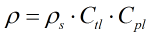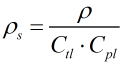# C019 – IP Paper 2/Table 54 Density Referral

## Description

This converts density values between standard conditions (1.01325 bar and 15°C) and other observed or line conditions.

Kelton calculation reference C019

FLOCALC calculation reference F029

KIMS calculation reference K210

### Ctl The correction for the pressure of the liquid is calculated using

“Report on the Development, Construction, Calculation and Preparation of the ASTM-IP Petroleum Measurement Tables (ASTM D 1250; IP 200)”: First edition 1960, Reprinted 1962 and 1968.(see C219)

### Cpl The correction for the temperature of the liquid is calculated using

Institute of Petroleum, Petroleum Measurement Paper No. 2; Guidelines for users of the petroleum measurement tables (API Std 2540; IP 200; ANSI/ASTM D 1250) September 1984.(see C223)

## Options

### Solve for

• Standard density

Calculate the standard density from the density at a given pressure and temperature

• Density

Calculate the density at a given pressure and temperature from the standard density

### Precision

• Full

This enables you to bypass the rounding methods outlined in the algorithms and use the full precision during the calculation

• Rounding

Select this option to follow the algorithms to the in the standard

### Vapour Pressure

• Include

This option allows the entry of the vapour equilibrium pressure, If this is not selected the value of 1 standard atmosphere (101325 Pa) is assumed.

## Calculations

### Density

When solving for density the temperature correction according to Table 54 is applied followed by the pressure correction according to IP Paper 2.### Standard Density

When solving for standard density an iterative calculation is performed making use of the Table 54 and IP Paper 2 algorithms. An initial estimate is taken for standard density based upon the entered density. This value is then fed into the Table 54 temperature correction algorithm to gain the Ctl. The estimate for standard density is then taken into the IP Paper 2 pressure correction algorithm to gain the Cpl. The Ctl and Cpl are then fed into the below formula to gain a new estimate of standard density. The process is repeated until standard density values converge to within 10-6.Back